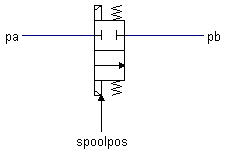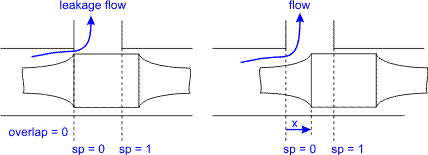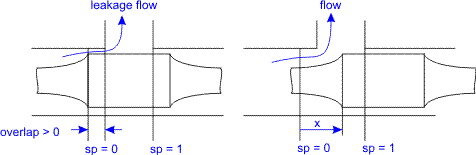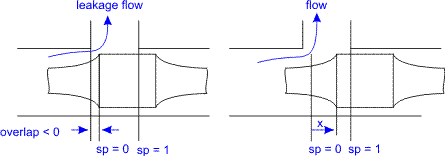﻿ 20-sim webhelp > Library > Iconic Diagrams > Hydraulics > Valves > Basic Valves > TwoTwoWayDirectionalValve

# TwoTwoWayDirectionalValve

Navigation:  Library > Iconic Diagrams > Hydraulics > Valves > Basic Valves >

# TwoTwoWayDirectionalValve## Library

Iconic Diagrams\Hydraulics\Valves\Basic Valves

## Use

Domains: Continuous. Size: 1-D. Kind: Iconic Diagrams (Hydraulics).

## DescriptionThis model describes a 2/2-way directional control valve with second order spool dynamics. The flow through the valve is described as laminar/turbulent flow through an orifice:

dp = pa.p - pb.p

phi = sign(dp) * Cd * A(sp) * sqrt( (2/rho) * abs(dp) ) + GLeak * dp;

Here Cd is the discharge coefficient which normally has a value between 0.55 and 0.7. A(sp) is the area of the orifice opening. A(sp) depends linearly on the spool position sp and varies between 0 (sp = 0) and the maximum area Amax (sp = 1). Gleak is the conductance of laminar leakage flow when the valve is closed. The relative opening of the spool valve is indicated by sp. For a closed valve the spool position (sp) is equal to zero and for an open valve the spool position (sp) is equal to 1.In the neutral spool position (sp = 0) the valve is just closed. A positive overlap indicates that the spool must travel a certain distance before the valve opens.A negative overlap indicates that the valve is already open in the neutral position.The overlap is indicated by the parameter overlap, which is given as a fraction of the spool position.

The spool position sp is a function of the input signal spoolpos:

sp = SO(f,d,discrete(spoolpos))

where SO is a second order transfer function to model the spool dynamics. The function is characterized by the bandwidth (f) and damping (d). The model acts as a directional valve (a valve which is either open or closed) because the input signal spoolpos is rounded to 0 or 1:

spoolpos < 0.5 => 0

spoolpos >= 0.5 => 1

The pressure at both ports has a lower limit which is equal to the vapour pressure. Therefore the actual equations used in this component are:

pa_lim = if pa.p < p_vapour then p_vapour else pa.p end;

pb_lim = if pb.p < p_vapour then p_vapour else pb.p end;

## Interface

 Ports Description pa, pb Both terminals of the valve. Causality fixed volume flow out pa fixed volume flow out pb Inputs spoolpos position of the spool valve spoolpos < 0.5 => valve is closed spoolpos >= 0.5 => valve is open Parameters rho GLeak Amax Cd overlap f d Mass density of the fluid [kg/m3]. Conductance of the laminar leakage flow [m3/s.Pa], GLeak >= 0. Maximum spool valve area when the valve is open [m2], Amax > 0. Discharge coefficient [], Cd > 0. The valve overlap in closed position [], -1 < overlap < 1. Bandwidth of the spool dynamics [Hz], f > 0. Damping of the spool dynamics [], d > 0.